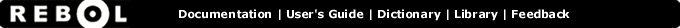# * - Function Summary

## Summary:

Returns the first value multiplied by the second.

* value1 value2

## Arguments:

value1 - The value1 argument. (must be: number pair char money time tuple)

value2 - The value2 argument. (must be: number pair char money time tuple)

## Description:

The datatype of the second value may be restricted to INTEGER or DECIMAL, depending on the datatype of the first value (e.g. the first value is a time).

 ``` print 123 * 10 1230```

 ``` print 12.3 * 10 123.0```

 ``` print 3:20 * 3 10:00```

## Related:

/ - Returns the first value divided by the second.
// - Returns the remainder of first value divided by second.
divide - Returns the first value divided by the second.
multiply - Returns the first value multiplied by the second.